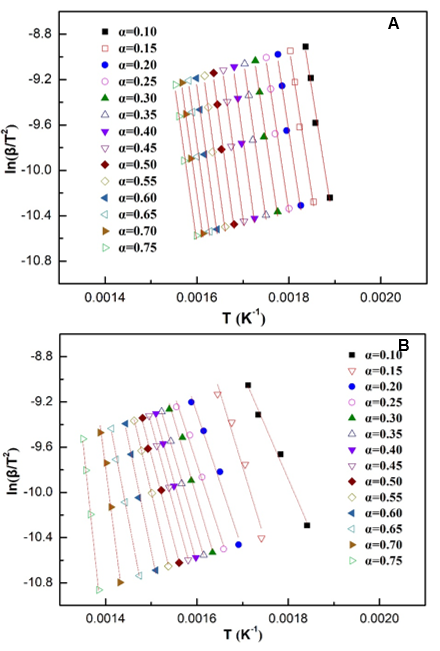Ma, H., Zhang, Y., Wang, L., Zhu, Z., Chen, Y., Wang, H., Deng, C., and Zhou, J. (2020). "Kinetic analysis of the pyrolysis of apricot stone and its main components via distributed activation energy mode," BioRes. 15(1), 1187-1204.

#### Abstract

The kinetics of pyrolysis of apricot stone and its main components, i.e., lignin, cellulose, and hemicellulose, were investigated via distributed activation energy mode. Experiments were done in a thermogravimetric analyzer at heating rates of 10, 20, 30, and 40 K·min-1 under nitrogen. The activation energy distribution peaks for the apricot stone, lignin, cellulose, and hemicellulose were centered at 246, 318, 364, and 170 kJ·mol-1, respectively. The activation energy distribution for the apricot stone slightly changed; lignin exhibited the widest distribution; and cellulose exhibited the highest activation energy at a conversion degree (α) of less than 0.75. At low pyrolysis temperatures (400 K to 600 K), the pyrolysis of hemicellulose was the main pyrolysis reaction. The apparent activation energy for the apricot stone mainly depended on the pyrolysis of hemicellulose and a small amount of lignin, and the activation energy was low in the early stage of pyrolysis. With the continuous increase in the pyrolysis temperatures (600 K to 660 K), the thermal weight loss of cellulose and lignin was intense. The apparent activation energy for the apricot stone mainly resulted from the pyrolysis of cellulose and lignin, and a higher activation energy was observed in the later stage of pyrolysis.

Kinetic Analysis of the Pyrolysis of Apricot Stone and its Main Components via Distributed Activation Energy Mode

Huanhuan Ma, Yimeng Zhang, Liangcai Wang, Zhengxiang Zhu, Yu Chen, Huilin Wang, Congjing Deng,* and Jianbin Zhou *

The kinetics of pyrolysis of apricot stone and its main components, i.e., lignin, cellulose, and hemicellulose, were investigated via distributed activation energy mode. Experiments were done in a thermogravimetric analyzer at heating rates of 10, 20, 30, and 40 K·min-1 under nitrogen. The activation energy distribution peaks for the apricot stone, lignin, cellulose, and hemicellulose were centered at 246, 318, 364, and 170 kJ·mol-1, respectively. The activation energy distribution for the apricot stone slightly changed; lignin exhibited the widest distribution; and cellulose exhibited the highest activation energy at a conversion degree (α) of less than 0.75. At low pyrolysis temperatures (400 K to 600 K), the pyrolysis of hemicellulose was the main pyrolysis reaction. The apparent activation energy for the apricot stone mainly depended on the pyrolysis of hemicellulose and a small amount of lignin, and the activation energy was low in the early stage of pyrolysis. With the continuous increase in the pyrolysis temperatures (600 K to 660 K), the thermal weight loss of cellulose and lignin was intense. The apparent activation energy for the apricot stone mainly resulted from the pyrolysis of cellulose and lignin, and a higher activation energy was observed in the later stage of pyrolysis.

Keywords: Apricot stone; Pyrolysis; Kinetics analysis; Distributed activation energy mode; Conversion degree

Contact information: a: Materials Science and Engineering College, Nanjing Forestry University, Nanjing 210037, China; b: Planning and Design Institute of Forest Products Industry, State Forestry and Grassland Administration, Beijing 100010, China;

* Corresponding authors: 15801407563@163.comzhoujianbin@njfu.edu.cn

INTRODUCTION

With the gradual substitution of conventional fossils, increased importance has been placed on the production of carbon-neutral, low-emission fuels from biomass renewable resources, which is a significant feedstock for the renewable production of fuels and chemicals (Gaurav et al. 2017; Akhtar et al. 2018). As the only renewable carbonaceous resource, biomass demonstrates the potential to produce heat, electricity, fuel, chemicals, and other products, and the utilization of biomass resources has attracted increasing attention (Tan et al. 2019; Usman et al. 2019).

Thermochemical conversion technology is regarded as a key technology that enables the conversion of biomass into high value-added chemicals and fuels. The main thermochemical processes include pyrolysis (Xu et al. 2019), combustion (Dorez et al. 2014), gasiﬁcation (Li et al. 2018), liquefaction (Wang et al. 2018), and carbonization (Li et al. 2008). Among these thermochemical pathways, pyrolysis, which involves the thermal decomposition of organics in the absence of oxygen, has been extensively developed as a promising platform to produce fuels and chemicals from various types of biomass (Cai et al. 2014). Lignocellulosic biomass is mainly composed of lignin, cellulose, and hemicellulose (Wang et al. 2016c). Lignin is typically composed of methoxylated phenylpropane units, which are polymerized by monolignols (p-hydroxyphenyl, guaiacyl, and syringyl units), and different ether bonds and carbon–carbon bonds (Jiang et al. 2010). In contrast to lignin, cellulose and hemicellulose exhibit relatively simple structures, only containing sugar units (such as pentoses and hexoses) and glycosidic bonds (Shen et al. 2010a).

Due to the complexity of the biomass pyrolysis reaction, it is extremely difficult to describe the entire reaction process and its interaction from the perspective of a microscopic process (Ranzi et al. 2008; Chen et al. 2014). To obtain relatively simple models to guide the process design, the laws of pyrolysis are typically explained by epigenetic characteristics. Pyrolysis kinetics is an important method used by graduate students to examine the pyrolysis characteristics and mechanism of solid substances such as biomass (Hu et al. 2016), coal (Bhagavatula et al. 2016; Coimbra et al. 2019), and other solid combustibles (Bartocci et al. 2019; Song et al. 2019). Thermal analysis serves as a high-precision analysis method for examining reaction kinetics, reflecting the rules and characteristics of pyrolysis from the perspective of solid biofuels (Ma et al. 2015; Carvalho and Tannous 2017; Zhou et al. 2018). The Kissinger–Akahira–Sunose method (Slopiecka et al. 2012; Ali et al. 2018), Flynn–Wall–Ozawa method ( Damartzis et al. 2011; Carvalho and Tannous 2017), Kissinger method (Viottoa et al. 2018), and distributed activation energy mode (DAEM) method (Zhang et al. 2014; De Caprariis et al. 2015) are widely employed for the kinetics analysis of the pyrolysis of biomass.

Apricot (Prunus armeniaca L.) is an important econo-ecological tree species in semi-arid areas of northern China, and the deep processing of its fruits affords its stone as the waste product, with an annual output of 400,000 to 500,000 tons. Apricot stone is an important raw material for preparing activated carbon (Jia et al. 2013; Yang et al. 2015; Marzbali et al. 2016), which is mainly composed of lignin, cellulose, hemicellulose, and other organic macromolecular components (Ali et al. 2011; Corbett et al. 2015). The chemical structures of these three components are different. The pyrolysis of apricot stone can be considered as the comprehensive performance of the pyrolysis reactions of the three components, and the pyrolysis process is relatively complex (Yang et al. 2007; Demiral and Kul 2014). Therefore, in the basic research of biomass pyrolysis, it is crucial to conduct in-depth research on the pyrolysis mechanism of raw materials and major components (Yan et al. 2019). Many previous studies on biomass components have been based on different model compounds, lignin, cellulose and hemicellulose isolated from biomass are closer to the natural form. In this study, the heat reaction of an apricot stone, as well as its components of lignin, cellulose, and hemicellulose, were examined by the DAEM, and the relationship between the pyrolysis activation energy and conversion degree was discussed. The results provided basic data and a theoretical basis for the efficient utilization of apricot stone biomass and research of the thermal reaction mechanism.

EXPERIMENTAL

Materials

Apricot stone raw material

Apricot stone was collected from the Hebei Province (China), milled, and screened to obtain a particle size of 100-mesh. Proximate analysis was tested according to ASTM D1102-84 (2013), ASTM E872-82 (2013), ASTM E871-82 (2013). The ultimate analysis was performed under the C, H, N, and S models using an elemental analyzer (Vario EL III; Elementar, Hanau, Germany), and oxygen was estimated by the difference method: O (%) = 100% C (%) H (%) N (%) S (%) Ash (%). Table 1 summarizes the results obtained.

Table 1. Proximate and Ultimate Analyses of the Apricot Stone (Dry Basis)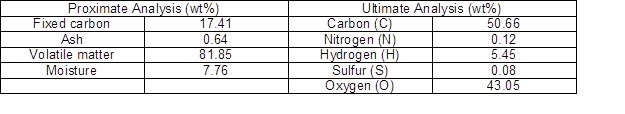The pretreated raw material of the apricot stone was extracted using a benzene–ethanol mixture (2/1, v/v) for 6 h. Next, this extracted material was subjected to vacuum drying in an oven at 50 C for 12 h, followed by grinding until all of the raw material was screened through a 140-mesh to extract lignin, cellulose, and hemicellulose.

Extraction of lignin

A 72% sulfuric acid solution was added to the pretreated raw material and it was stirred sufficiently at 20 C for 2.5 h. The solution was filtered, and the filtrate liquor was boiled and subjected to reflux conditions with distilled water for 4 h. The liquor was left to stand for 30 min before filtering, and the filtered residue was repeatedly washed with hot water until a neutral pH was attained. The sample obtained after drying was the apricot stone lignin according to TAPPI T222 (2002), also referred to as Klason lignin (30.42% yield).

Extraction of cellulose

First, 260 mL of distilled water, 3 g of sodium chlorite, and 2 mL of acetic acid were added into 8 g of the pretreated raw material and subjected to oscillation twice at 75 C for 1 h. After the reaction was completed, the reaction was immediately placed in ice water, followed by filtration. Then, the residue was washed with distilled water until a neutral pH was attained. Next, the residue was washed thrice with acetone, air-dried in a fume hood, and dried in a drying oven at 50 C for 12 h. The sample obtained was apricot stone holocellulose.

First, 24 wt% of KOH and 2 wt% of H3BO3 at a solid–liquid ratio of 1:20 were added into 10 g of holocellulose, followed by vibration in a constant-temperature water bath at 20 C for 2 h. After the reaction was completed, it was filtered, and the residue was washed with distilled water until a neutral pH was attained (Stefanidis et al. 2014). The sample obtained after freeze-drying the residue was apricot stone cellulose (24.6% yield).

Extraction of hemicellulose

First, a 17 wt% NaOH solution was used to extract apricot stone holocellulose at 20 C for 2 h before filtration. The filtered liquor was neutralized by 6 mol·L1 acetic acid until its pH was 5.5, followed by the addition of a 95 wt% ethanol solution to allow for the complete precipitation of hemicellulose, followed by centrifugation, filtration, and freeze-drying (Stefanidis et al. 2014). The sample obtained after freeze-drying the residue was apricot stone hemicellulose (29.4% yield).

Methods

The thermal properties of the apricot stone and its main components were examined using a thermal gravimetric analyzer (TGA Q5000; TA Instruments, New Castle, DE, USA). All samples were individually heated from room temperature to 1073 K at different heating rates of 10, 20, 30, and 40 K·min1. The weight of each experimental sample was 15 mg, the carrier gas was high-purity nitrogen, and the flow rate was 70 mL·min1.

Kinetic Studies

The DAEM was first proposed in 1942 (Vand 1943), and was gradually applied for the pyrolysis of fossil fuels. The DAEM is applied based on the hypothesis of an infinite parallel reaction and activation energy distribution. It has been widely applied to the kinetics analysis of some complex reaction systems (Lin et al. 2018; Ren et al. 2018). Biomass pyrolysis comprises multiple reactions. Let the total volatilization of the i of the reaction be Vi* and the volatilization amount up to time t be Vi. According to the two assumptions of the DAEM model, the kinetics equation of reaction i is expressed as follows, where k represents kinetic constant (mol·s1), A represents the pre-exponential factor, R represents universal gas constant (8.314 J·mol1·K1), and T is absolute temperature (K):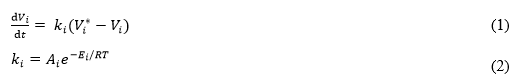By integrating both sides of the transformation Eq. 1,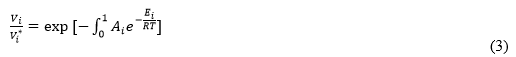when the reaction number of the system is 1, the above model is a first-order model reaction. In fact, the composition of biomass, such as an apricot stone, is extremely complex, and many reactions are possible during pyrolysis. The corresponding reaction quantities in the DAEM model can be expressed by differential calculus; namely, dV replaces Vi, dV* replaces Vi*, and dVdepends on the activation energy distribution: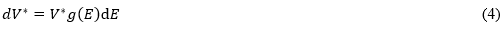According to the above equation, the distributed activation energy function satisfies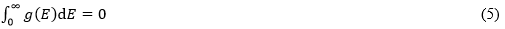The differential transformation of Eq. 4 is carried out, and Eqs. 6 and 7 are as follows: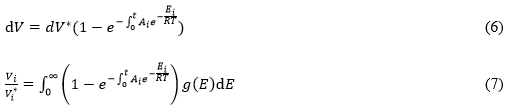Non-isothermal thermal analysis is adopted, and Eq. 8 is characterized by the mass fraction of the residual matter analyzed by TGA and the mass fraction of the residual matter at the end of pyrolysis, in which w represents the initial mass (mass%), and wf represents the final residual mass (mass%):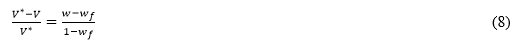The Miura integral method was employed to examine the activation energy distribution characteristics of the apricot stone, lignin, cellulose, and hemicellulose. According to the Miura integral principle, the Arrhenius equation is expressed as follows: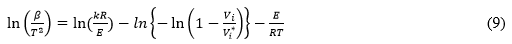The TGA method was employed to measure the relationship between the conversion degree α (V/V*) and T at different heating rates β (i.e., 10, 20, 30, and 40 K·min1), and, by using the TGA curve data, ln(β/T2) was plotted as a function of 1/T. The Arrhenius line was plotted using the same α value at different heating rates through the Origin software (Origin, OriginLab, 8.5, Massachusetts, USA); according to the slope of the line, the activation energy E under the conversion rate can be calculated (Wang et al. 2012).

RESULTS AND DISCUSSION

TGA of the Apricot Stone

The TG and DTG curves of the apricot stone exhibited the same change trends at different heating rates (Fig. 1). The main pyrolysis temperature range was 475 K to 775 K, and the weight loss was ~76%. The heating rates were 10, 20, 30, and 40 K·min1 and the pyrolysis temperature was 1075 K; the pyrolysis yields of solid products were 18.4%, 18.2%, 18.2%, and 17.1%, respectively.

With the increase in the heating rate, the TG curves shifted to the right, indicating that instantaneous pyrolysis weightlessness decreased at the same pyrolysis temperature. It was mainly affected by the heat and mass transfer. From the DTG curves of the apricot stone, the pyrolysis interval consisted of two peaks. The temperatures associated with the first peak were 551.5 K, 564.2 K, 571.1 K, and 573.1 K, and their corresponding weight loss rates were 5.26 wt%·min1, 10.69 wt%·min1, 15.85 wt%·min1, and 20.22 wt%·min1 at heating rates of 10 K·min1, 20 K·min1, 30 K·min1, and 40 K·min1, respectively. The temperatures associated with the second peak were 620.3 K, 630.1 K, 635.5 K, and 638.1 K, respectively, and their corresponding weight loss rates were 7.50 wt%·min1, 14.57 wt%·min1, 21.54 wt%·min1, and 27.76 wt%·min1 at heating rates of 10 K·min1, 20 K·min1, 30 K·min1, and 40 K·min1, respectively.

As the heating rate increased, the temperature of the two positions of peaks increased, and the maximum weight loss rate increased dramatically (Ahmad et al. 2018; Saikia et al. 2018).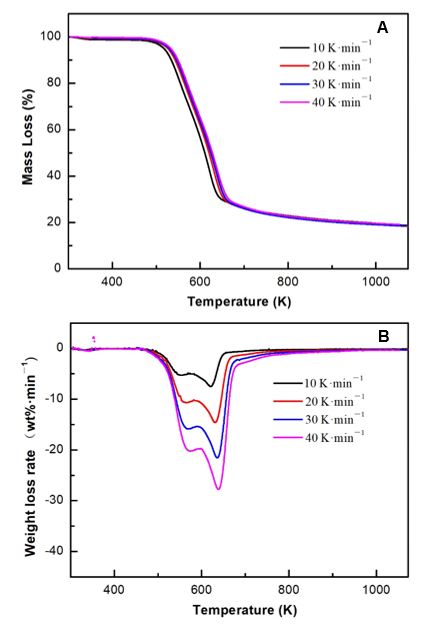Fig. 1. TG (a) and DTG (b) curves of the apricot stone

TGA of Apricot Stone Lignin

The TG and DTG curves of the apricot stone lignin exhibited the same change trends at different heating rates (Fig. 2). The main pyrolysis temperature range was relatively wide (500 K to 1000 K), and the weight loss was 60% to 66%. The heating rates were 10 K·min1, 20 K·min1, 30 K·min1, and 40 K·min1; the pyrolysis temperature was 1075 K, and the pyrolysis yields of solid products were 38.5%, 37.0%, 35.7%, and 32.2%, respectively. The peak temperatures corresponding to the DTG curve moved to the high-temperature direction; the maximum weightlessness temperatures were 624.9 K, 641.5 K, 654.2 K, and 661.1 K, and the maximum weightlessness rates were 5.33 wt%·min1, 9.59 wt%·min1, 14.18 wt%·min1, and 20.75 wt%·min1, respectively. The maximum weightlessness temperatures of the apricot stone lignin were greater than those of the apricot stone, while the maximum weightlessness rates were lower (Shen et al. 2010b; Stefanidis et al. 2014).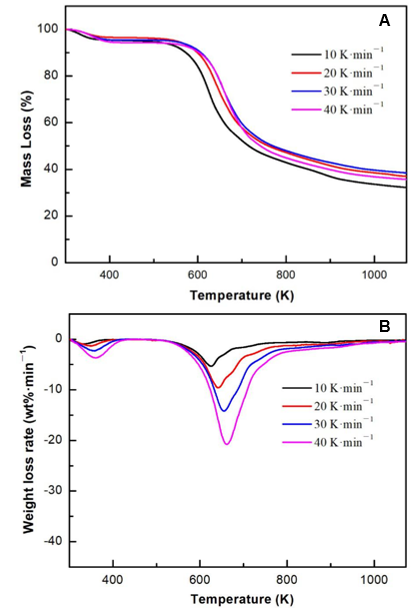Fig. 2. TG (a) and DTG (b) curves of the apricot stone lignin

TGA of Apricot Stone Cellulose

A narrow pyrolysis temperature range for apricot stone cellulose (520 K to 775 K) was observed (Fig. 3). With the increase in the heating rate, due to the effect of heat-transfer hysteresis, the positions of the TG curve and maximum weight loss rate shifted to the high-temperature side. With the increase in the heating rate from 10 K·min1 to 40 K·min1, the maximum weight loss temperature gradually increased from 623.17 K to 648.39 K, and the weight loss rate increased from 14.04 wt%·minto 41.11 wt%·min1. The macromolecule chain of apricot stone cellulose was neat, which meant that the polymer chains break first (Soni et al. 2015). The dissociation energy of glycosides that were between the adjacent ring bonds was similar, and as the initial pyrolysis temperature of weightlessness gradually increased, the DTG curve exhibits sharp weightlessness peaks (Burhenne et al. 2013).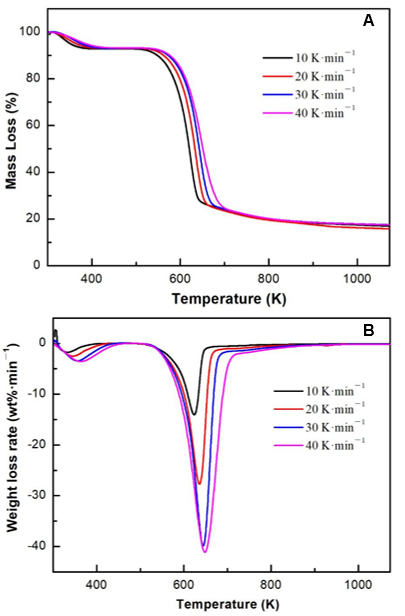Fig. 3. TG (a) and DTG (b) curves of apricot stone cellulose

TGA of Apricot Stone Hemicellulose

The pyrolysis temperature range of apricot stone hemicellulose was 475 K to 700 K (Fig. 4). With the increase in the heating rate, the starting and ending temperatures of each stage slightly shifted to the high-temperature side, and the main reaction interval also slightly increased because of the presence of side chains in the molecular structure of apricot stone hemicellulose, which were easily broken at lower temperature. After the decomposition of the side chain, the main reaction tended to be polymer-chain depolymerization and intramolecular dehydration condensation. The heating rates were 10 K·min1, 20 K·min1, 30 K·min1, and 40 K·min1, and the weight loss rate extremum positions were 568.7 K, 581.7 K, 587.9 K, and 592.6 K, respectively, in the DTG curve. These temperatures were the minimum temperatures of the maximum weight loss temperature for the pyrolysis of apricot stone and its components in different heating rates, suggesting that apricot stone hemicellulose was the most unstable component (Wang et al. 2016b).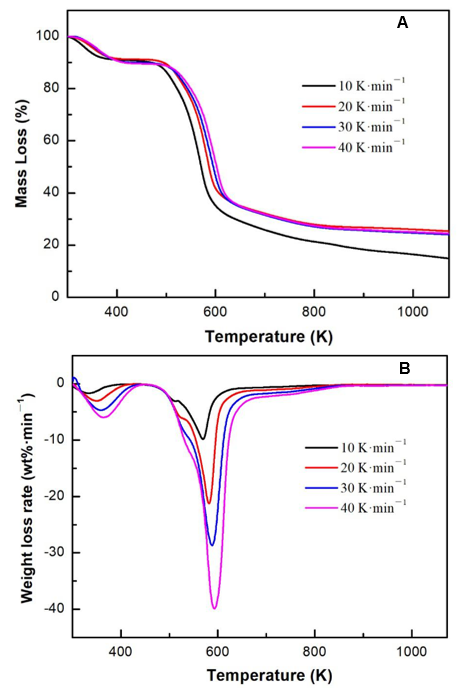Fig. 4. TG (a) and DTG (b) curves of apricot stone hemicelluloses

Kinetics Analysis

Arrhenius line

The DAEM method was employed to understand the relationship between T and α at different temperature increase rates during pyrolysis. According to the TG curve data, ln (β/T2) was plotted as a function of 1/T, and the Arrhenius line was fitted with the same α value at different heating rates. According to the slope of the line fitting equation, the activation energy (E) that corresponded to the conversion rate was obtained. Figure 5 shows the Arrhenius plots of the apricot stone, lignin, cellulose, and hemicellulose at heating rates of 10 K·min1, 20 K·min1, 30 K·min1, and 40 K·min1 as a function of α.